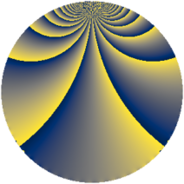# Properties

 Label 3630.2.jLevel $3630$ Weight $2$ Character orbit 3630.j Rep. character $\chi_{3630}(1937,\cdot)$ Character field $\Q(\zeta_{4})$ Dimension $436$ Sturm bound $1584$

# Related objects

## Defining parameters

 Level: $$N$$ $$=$$ $$3630 = 2 \cdot 3 \cdot 5 \cdot 11^{2}$$ Weight: $$k$$ $$=$$ $$2$$ Character orbit: $$[\chi]$$ $$=$$ 3630.j (of order $$4$$ and degree $$2$$) Character conductor: $$\operatorname{cond}(\chi)$$ $$=$$ $$15$$ Character field: $$\Q(i)$$ Sturm bound: $$1584$$

## Dimensions

The following table gives the dimensions of various subspaces of $$M_{2}(3630, [\chi])$$.

Total New Old
Modular forms 1680 436 1244
Cusp forms 1488 436 1052
Eisenstein series 192 0 192

## Trace form

 $$436q + 4q^{3} - 4q^{6} + 4q^{7} + O(q^{10})$$ $$436q + 4q^{3} - 4q^{6} + 4q^{7} - 4q^{10} - 4q^{12} - 16q^{13} + 16q^{15} - 436q^{16} + 8q^{18} - 8q^{21} + 16q^{25} + 4q^{27} + 4q^{28} - 12q^{30} - 24q^{31} + 12q^{36} - 40q^{37} + 8q^{40} - 28q^{42} - 24q^{43} + 24q^{45} + 32q^{46} - 4q^{48} + 40q^{51} + 16q^{52} + 16q^{57} + 36q^{58} - 12q^{60} + 8q^{61} - 44q^{63} + 16q^{67} - 4q^{70} - 8q^{72} + 36q^{73} - 44q^{75} + 16q^{76} - 16q^{78} - 68q^{81} - 48q^{82} - 8q^{85} + 84q^{87} - 24q^{90} + 96q^{93} + 4q^{96} + 100q^{97} + O(q^{100})$$

## Decomposition of $$S_{2}^{\mathrm{new}}(3630, [\chi])$$ into newform subspaces

The newforms in this space have not yet been added to the LMFDB.

## Decomposition of $$S_{2}^{\mathrm{old}}(3630, [\chi])$$ into lower level spaces

$$S_{2}^{\mathrm{old}}(3630, [\chi]) \cong$$ $$S_{2}^{\mathrm{new}}(30, [\chi])$$$$^{\oplus 3}$$$$\oplus$$$$S_{2}^{\mathrm{new}}(165, [\chi])$$$$^{\oplus 4}$$$$\oplus$$$$S_{2}^{\mathrm{new}}(330, [\chi])$$$$^{\oplus 2}$$$$\oplus$$$$S_{2}^{\mathrm{new}}(1815, [\chi])$$$$^{\oplus 2}$$